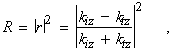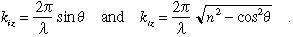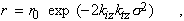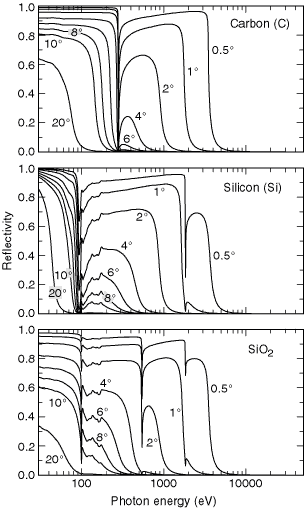## Section 4.2   Specular Reflectivities for Grazing-Incidence MirrorsThe specular reflectivity of six common materials is given in Figs. 4-4 and 4-5 for photon energies between 30 eV and 30 keV. The reflectivity for a perfectly smooth surface and for s-polarization is(1)

whereThe grazing angle q is measured from the plane of the mirror surface. The normal components of the incident and transmit­ted wave vectors are kiz and ktz, respectively. The complex index of refraction n is obtained from the average atomic scat­tering factor of the material, as described in Section 1.7 and in Ref. 1.

The effect of high-spatial-frequency roughness on the reflection coefficient of an interface can be approximated by the multiplicative factor(2)

where r0 is the complex reflection coefficient of a perfectly smooth interface and s is the rms roughness. For updated values of the atomic scattering factors and for on-line reflectivity calculations, see http://www-cxro.lbl.gov/ optical_constants/.

REFERENCE

1.      B. L. Henke, E. M. Gullikson, and J. C. Davis, “X-Ray Interactions: Photoabsorption, Scattering, Transmission, and Reflection at E = 50–30,000 eV, Z = 1–92,” At. Data Nucl. Data Tables 54, 181 (1993).Fig. 4-4.    Specular reflectivities of carbon (r = 2.2 g/cm3), silicon (r = 2.33 g/cm3), and silicon dioxide (r = 2.2 g/cm3). The reflectivity is calculated for s-polarization at grazing angles of 0.5, 1, 2, 4, 6, 8, 10, and 20 degrees.Fig. 4-5.    Specular reflectivities of nickel (r = 8.90 g/cm3), ruthenium (r = 12.41 g/cm3), and gold (r = 19.3 g/cm3). The reflectivity is calculated for s-polariza­tion at grazing angles of 0.5, 1, 2, 4, 6, 8, 10, and 20 degrees.